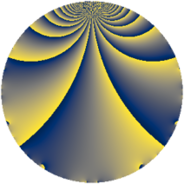# Properties

 Label 1045.2.eLevel $1045$ Weight $2$ Character orbit 1045.e Rep. character $\chi_{1045}(1044,\cdot)$ Character field $\Q$ Dimension $116$ Sturm bound $240$

# Related objects

## Defining parameters

 Level: $$N$$ $$=$$ $$1045 = 5 \cdot 11 \cdot 19$$ Weight: $$k$$ $$=$$ $$2$$ Character orbit: $$[\chi]$$ $$=$$ 1045.e (of order $$2$$ and degree $$1$$) Character conductor: $$\operatorname{cond}(\chi)$$ $$=$$ $$1045$$ Character field: $$\Q$$ Sturm bound: $$240$$

## Dimensions

The following table gives the dimensions of various subspaces of $$M_{2}(1045, [\chi])$$.

Total New Old
Modular forms 124 124 0
Cusp forms 116 116 0
Eisenstein series 8 8 0

## Trace form

 $$116 q - 120 q^{4} - 2 q^{5} + 100 q^{9} + O(q^{10})$$ $$116 q - 120 q^{4} - 2 q^{5} + 100 q^{9} - 2 q^{11} + 112 q^{16} - 40 q^{20} + 2 q^{25} - 24 q^{26} - 160 q^{36} + 4 q^{44} - 18 q^{45} + 88 q^{49} - 29 q^{55} - 208 q^{64} - 72 q^{66} + 108 q^{80} + 84 q^{81} - 98 q^{99} + O(q^{100})$$

## Decomposition of $$S_{2}^{\mathrm{new}}(1045, [\chi])$$ into newform subspaces

The newforms in this space have not yet been added to the LMFDB.